##### Abstract

Mirage is a beautiful natural phenomena where a light ray coming down at an inclination to the vertical turns up side down due to gradient in refractive index. It can be easily demonstrated in a laboratory by making a salt solution with a concentration gradient. In this article, we describe our experiment on forming a Mirage like phenomena and analyze the path using Snell's law and also using Fermat's principal.

##### I. Introduction

On a hot summer day, the sand in desert area becomes very hot and creates a temperature gradient in the air above it. Accordingly, the density of air also acquires a gradient, decreasing as one goes up and correspondingly the refractive index increases gradually as one goes up. Light rays starting obliquely from some object, gradually turn away from the local normal (vertical) as it moves from optically denser to rarer layers of air and at some stage become horizontal. Interestingly, it does not continue in this horizontal layer as one may expect from a naive argument that it is no more “falling” on a layer with different refractive index. Rather it turns upwards and keeps deviating. If an observer is stationed at appropriate position, she/he may observe the virtual image of the object as if the sand is behaving like a plane mirror.

Most of the articles and textbooks rightly describe this phenomena as a result of Fermat’s principle or of refraction in a medium with gradually changing refractive index (1-3). However a quantitative analysis leading to the equation of the path of a light ray in such a medium is seldom attempted. In some of the popular sites and also in some of the textbooks including NCERT book, it is erroneously mentioned that in mirage, at some stage the angle of incidence becomes larger than the critical angle and total internal reflection makes the light ray turn upwards (4).

Though the author has not experienced Mirage in a desert but it is very common to observe this phenomena while driving on a highway during peak summer days. Not only that the road appears wet, one can see images of headlights of the vehicles coming from opposite side if they are turned on. In the following, we describe our experiments of light following a curved path and turning upside down in a salt solution and then derive the equation of the path from Snell’s law, establishing clearly that refraction can turn the light ray up side down.

##### II. Experiment

About 30 g of table salt was dissolved in about 100 ml of water and it was placed in a transparent cubical container. Fresh water was taken in a syringe with the needle removed. It was held with the nozzle touching one of the walls of the container and the piston was gently moved in, injecting the water over the salt solution very slowly. About 50 ml of water was poured this way in the container. Care was taken not to vibrate or disturb the container in any other way.

Because of the density differences, fresh water tends to float on the salt solution but because of the thermal diffusion some mixing occurs at the interference. In a minute or so things stabilize and a concentration gradient is formed at the junction of solution and water, extending to several milli meters which stays for reasonably long periods of time. The refractive index of a salt solution increases with the increase in salt concentration and hence a refractive index gradient is established in the interface region.

A laser beam is sent through the solution in nearly horizontal direction. If the beam is close to the bottom, it is seen to go in a straight path. Same is the case when the beam is sent near the top. But if the beam is sent in the interface region, distinct curving is seen in the beam.

In Figure-1 we show the actual photograph when the laser beam is sent into the interface region with a small angle made with the horizontal direction. Thus the beam is risen up in the beginning, it gradually bends in the solution, becomes horizontal at some stage and then turns down again.

The order of bending is opposite to the atmospheric mirage which is natural as the refractive index gradually decrease as one goes up in the current set up.

##### 1 From Snell’s law

To analyze the path of the light beam, we use Snell’s law which is conventionally written as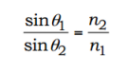Or
n1 sin θ1= n2 sin2
(1)

where the symbols have their standard meaning (Figure-3). In our case, the refractive index varies continuously and the appropriate from of Snell’s law (i) is

n sinθ= constant.

With the coordinate system shown in Figure 2, let us model the variation of refractive index to be linear and write

n=n0(1+ αx )

where n0 is the refractive index at x = 0 where the beam becomes horizontal.

At any general point P on the path, the normal to the layers is along the x-axis and so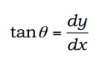(2)

At x = 0, n= n0 and θ=π/2. Applying Snell’s law for points P and O,

n0(1+αx)sinθ=n0

Or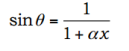(3)

Eliminating θ from Equations (2) and (3),Investigating the path close to x = 0, and also using the fact that the refracting index of salt solution is only slightly more than of water (α small), one can use the approximation αx << 1 and write

Or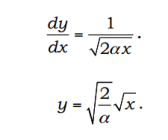(4)

This is a standard equation of a parabola with the x-axis as the axis of the parabola. This shows that obeying the law of refraction, the path of the light beam close to the point where it becomes horizontal, must be close to a parabola opening downwards.

##### 2 Verification using Fermat’s Principle

Take two points A and B in the same horizontal layer (Figure-3). Let AB=2y0. It should be possible to use calculus of variation and Euler-Lagrange equations to derive the path of the light beam requiring that the time taken by the light beam should be least among the neighboring paths. However we will not attempt for that and will be satisfied to show that among the parabolic paths from A to B, the one given by equation (iv) corresponds to the least time. These parabolas will include the ones opening upwards.

Consider a parabola ACB with the vertex C at (-b, 0). Thus b is the variable parameter and different values of b correspond to different parabolas. If b is negative, the parabola will open upwards. The value b = 0 corresponds to light going parallel to the layer without bending. The equation of the parabola isThis can be easily verified as it passes through A, B and C

Thus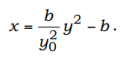Or,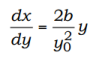Or,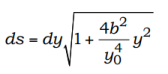Time taken by the light to cross this distance is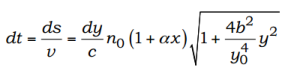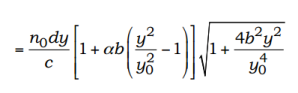The time to go from A to B is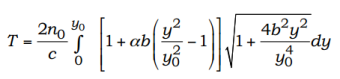As the refractive index varies slowly, αb << 1. Also with small overall difference in refractive index, b << y0. With these approximations,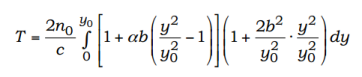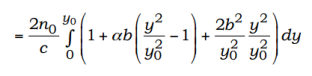We have retained terms up to the order of b3. The value of b which makes this time least is given by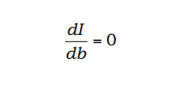Or,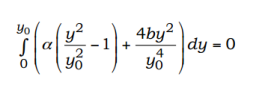Or,Or,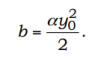Thus the equation of the parabola corresponding to the least time is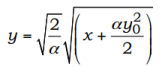If the shift the origin to the vertex, the above equation becomeswhich is the same as that derived using Snell’s law (Equation 4)

##### IV. Concluding Remarks

The phenomenon of mirage has been constructed in the laboratory using an inhomogeneous salt solution prepared carefully to have a concentration gradient in vertical direction. A light beam sent into the system obliquely from high concentration side to low concentration side indeed turns upside down and returns in the high concentration side.

Analytic equation of light path is derived from Snell’s law, nsinθ = constant , and is found to give correct shape as observed in the experiment. For small part near the turning point, this path is approximately parabolic. An analysis using Fermat’s principle reconfirms this parabolic path.

##### References
• 1. Edwin Meyer, Scientific American, vol. 289, November 2003

• 2. Paul G Hewitt, Conceptual Physics,3rd Ed, 10th Ed., Pearson Education and Dorling Kindersley, New Delhi p. 537

• 3. Paul A Tippler and Gene Mosca, Physics for Scientists and Engineers, W H Freeman, p. 2067

• 4. Physics textbook for class XII, NCERT, New Delhi, p. 321